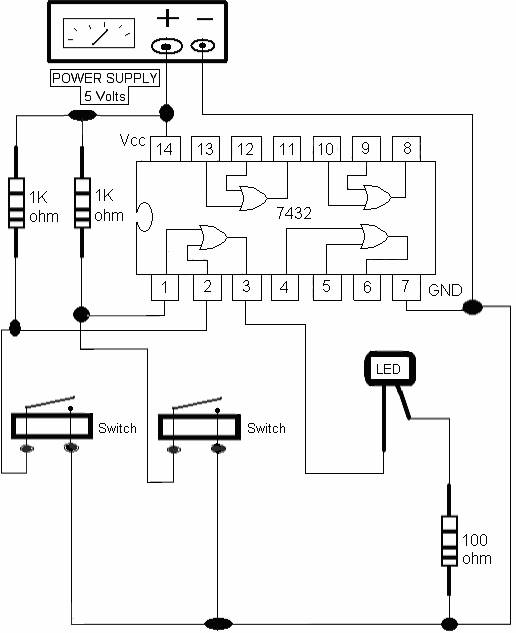OR GATE: OR gate has two inputs and one output. The symbol for OR gate

is Shown in figure 1.3 .This gate works based on the input/output voltage in

table 1.2.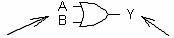Inputs (A and B)                         Output (Y)

Figure 1.3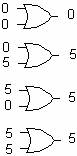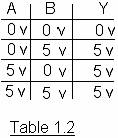OR gate is available in a six-pack in an IC chip.

The type number for OR gate is 7432.

The pin configuration of IC 7432 is shown in figure 1.4.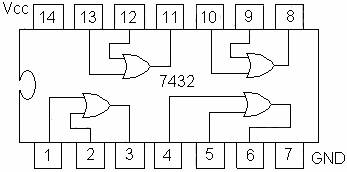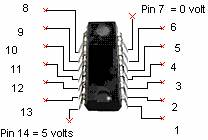Figure 1.4

Lab 1.2    Part One

Set up the circuit and verify table 1.2 (software).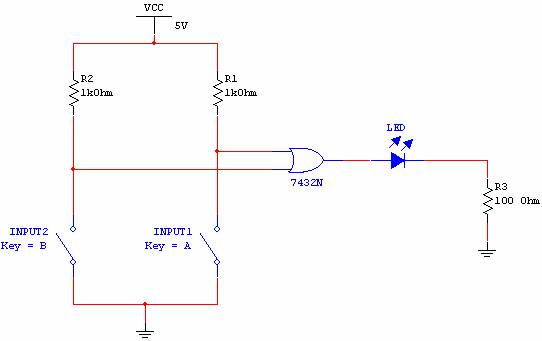Note:  When the switch is OPEN, this means the input is 5 volts (HIGH).

When the switch is CLOSED, this means the input is 0 volts (LOW).

1. When either input1 or input2 is 5 volts (Switch is OPEN), the output of OR gate is

5 volts (HIGH) and the LED is ON.

1. When both inputs 1 and 2 are 5 volts (Switch is OPEN), the output of OR gate is

5 volts (HIGH) and the LED is ON.

1. When both inputs 1 and 2 are 0 volts (Switch is CLOSED), the output of OR gate is 0 volts (LOW) and the LED is OFF.

Lab 1.2    Part Two

Set up the circuit diagram on page 6 using the following parts: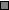5 Volts Power supplyWire, 22 GaugeOne IC 7432Two SPST switchesTwo 1K ohm Resistors (1/4 W)One Red LEDOne 100 ohm Resistor (1/4 W)